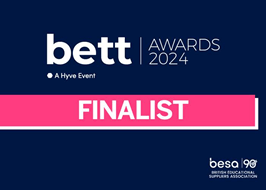# Energy bill calculatorCalculate an energy bill from meter readings to display on a smart meter.

With the invention of the dynamo in 1861, electrical energy could be generated in large amounts. The first mass application of electricity was lighting. When this new product – electrical energy – started to be sold, it was obvious that the cost had to be determined. The earliest meter was Samual Gardiner’s (USA) lamphour meter patented in 1872. It measured the time during which energy was supplied to the load, as all the lamps connected to this meter were controlled by one switch. Subdividing lighting circuits became practical with the introduction of Edison’s light bulb, and this meter became obsolete.

## Make

Write a program that calculates the cost of energy from a previous and current meter reading. The kilowatt hours is the total units_used between the two readings multiplied by 1.022 multiplied by the calorific_value divided by 3.6. The cost in pounds sterling is 0.0284 multiplied by kilowatt hours.

Use this boilerplate code as a starting point:

### Success Criteria

Remember to add a comment before a subprogram to explain its purpose.

#### Create a subprogram called `energy_cost` that:

3. Calculates the kilowatt hours as units_used * 1.022 * (calorific_value / 3.6).
4. Calculates the cost as 0.0284 * kilowatt hours
5. Returns the cost as an integer.

#### Complete the `main program` so that:

1. The user can input the previous meter reading as a four digit integer.
2. The user can input the current meter reading as a four digit integer.
3. The calorific value is set to be 39.3.
4. The cost is calculated using the subprogram.
5. The cost is output.

#### Typical inputs and outputs from the program would be:

Enter the previous meter reading: 2022

Enter the current meter reading rounded down: 2305

Cost is £ 89

Enter the previous meter reading: 7666

Enter the current meter reading rounded down: 8241

Cost is £ 182

#### Knowledge Organiser

Use these resources as a reference to help you meet the success criteria.

## Evaluate

Run the unit tests below to check that your program has met the success criteria.

Enter the previous meter reading: 2202

Enter the current meter reading rounded down: 2305

Cost is £ 32

Enter the previous meter reading: 7324

Enter the current meter reading rounded down: 8876

Cost is £ 491

Check that you have used comments within the code to describe the purpose of subprograms.

In partnership with